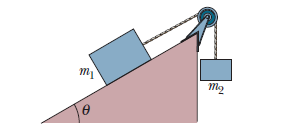# Problem: A block of mass m1 = 3.70 kg on a frictionless plane inclined at angle θ = 30.0° is connected by a cord over a massless,  frictionless pulley to a second block of mass m2 = 2.30 kg (Fig. 5-52). Find(a) the magnitude of the acceleration of each block,(b) the direction of the acceleration of the hanging block, (c) the tension in the cord.

###### FREE Expert Solution
80% (388 ratings)
###### Problem Details

A block of mass m1 = 3.70 kg on a frictionless plane inclined at angle θ = 30.0° is connected by a cord over a massless,  frictionless pulley to a second block of mass m2 = 2.30 kg (Fig. 5-52). Find
(a) the magnitude of the acceleration of each block,
(b) the direction of the acceleration of the hanging block,
(c) the tension in the cord.